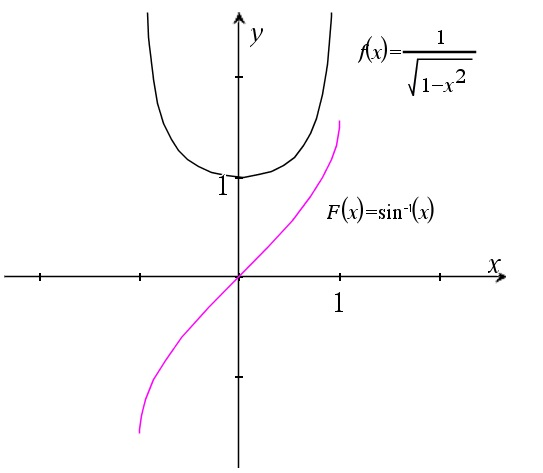# The integral of 1/sqrt(1-x^2) dx

Up a level : Integrals
Previous page : The integral of sin(x)cos(x)dx
Next page : The integral of 1/sqrt(x^2-1) dxLet us find$\int {\frac{{dx}}{{\sqrt {1 - {x^2}} }}}$

in a couple of different ways.

As an antiderivative$y = {\sin ^{ - 1}}x$

This may be rewritten as us$\sin y = x$

Now we take let us differentiate this with respect to x, remembering that y is a function of x. This means that we need to apply the chain rule. We get$\cos (y)y' = 1$

or$y' = \frac{1}{{\cos (y)}} = \frac{1}{{\cos ({{\sin }^{ - 1}}x)}}$

But$\cos ({\sin ^{ - 1}}x) = \sqrt {1 - {x^2}}$

This we can see from looking at the unit circle below.The arcsine of x gives us an angle, and the sine of that gives us the value along the horizontal axis, and in a unit circle the vertical and horizontal values are connected through the Pythagoras’s theorem to give the above result. This gives us that$({\sin ^{ - 1}}x)' = \frac{1}{{\sqrt {1 - {x^2}} }}$

and thus that$\int {\frac{{dx}}{{\sqrt {1 - {x^2}} }}} = {\sin ^{ - 1}}x + C$

Below is a graph of the integrand vs. the integral.By the substitution method

Now suppose we did not know this above derivative, then we could find the integral by doing the substitution$\left| \begin{gathered} x = \sin y \hfill \\ dx = \cos y\;dy \hfill \\ \end{gathered} \right.$

This because we may recognize that we then can use the trigonometric identity${\sin ^2}\theta + {\cos ^2}\theta = 1$

We get$\begin{gathered} \int {\frac{{\cos y\;dy}}{{\sqrt {1 - {{\sin }^2}y} }}} = \int {\frac{{\cos y\;dy}}{{\cos y}}} \hfill \\ \quad = \int {dy} = y + C = {\sin ^{ - 1}}x + C \hfill \\ \end{gathered}$

as before.

The above generalized a bit

Now if we have$\int {\frac{{dx}}{{\sqrt {{a^2} - {x^2}} }}}$

then we can pull out a factor of a from the square root to get$\int {\frac{{dx}}{{a\sqrt {1 - {{\left( {\frac{x}{a}} \right)}^2}} }}}$

If we now let$\left| \begin{gathered} w = x/a \hfill \\ dw = dx/a \hfill \\ adw = dx \hfill \\ \end{gathered} \right.$

we get$\begin{gathered} \int {\frac{{adw}}{{a\sqrt {1 - {w^2}} }}} = \int {\frac{{dw}}{{\sqrt {1 - {w^2}} }}} \hfill \\ \quad = {\sin ^{ - 1}}w + C = {\sin ^{ - 1}}\frac{x}{a} + C \hfill \\ \end{gathered}$

This we can get directly if we apply the rule$\int {f(px + q)dx = \frac{{F(px + q)}}{p} + C}$

to get$\begin{gathered} \int {\frac{{dx}}{{\sqrt {{a^2} - {x^2}} }}} = \int {\frac{{dx}}{{a\sqrt {1 - {{\left( {\frac{x}{a}} \right)}^2}} }}} \hfill \\ \quad = \frac{{\;\frac{1}{a}\;}}{{\;\frac{1}{a}\;}}{\sin ^{ - 1}}\frac{x}{a} = {\sin ^{ - 1}}\frac{x}{a} + C \hfill \\ \end{gathered}$Up a level : Integrals
Previous page : The integral of sin(x)cos(x)dx
Next page : The integral of 1/sqrt(x^2-1) dxLast modified: May 18, 2019 @ 11:01